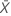# Methods and formulas for Attribute Gage Study (Analytic Method)

Select the method or formula of your choice.

## Bias

Bias is calculated as the difference between the known standard value of a reference part and the observed average measurement.

When the actual measurement is unknown, Attribute Gage Study (Analytic Method) estimates the bias as follows:
Point estimate of bias with a lower tolerance limit
bias = lower limit + intercept / slope
Point estimate of bias with an upper tolerance limit
bias = upper limit + intercept / slope

The intercept and slope for both formulas are from the fitted line on the probability plot.

Minitab regresses the z–score Φ-1(Prob (Acceptance)) on reference values XT to calculate the intercept and slope.

## Pre-adjusted repeatability

Pre-adjusted repeatability is the repeatability that is calculated before adjusting for over-estimation.

### Formula

Minitab estimates the pre-adjusted repeatability by:

### Notation

TermDescription
XTrepresents the estimated reference values at the 0.995 and 0.005 probabilities of acceptance, which are calculated from the fitted line on the probability plot.

## Repeatability

Repeatability is the amount of variation in the measurement system that is from the gage. An attribute gage study regresses the probabilities of acceptance on the reference values to obtain repeatability.

Pre-adjusted repeatability is the repeatability that is calculated before adjusting for over-estimation. Minitab divides estimates of repeatability by the adjustment factor 1.08 to calculate the adjusted repeatability.

### Formula

Minitab estimates the repeatability by:

### Notation

TermDescription
XTrepresents the estimated reference values at the 0.995 and 0.005 probabilities of acceptance, which are calculated from the fitted line on the probability plot.

The denominator, 1.08, is the adjustment factor given by the Automotive Industry Action Group1 Minitab uses the adjusted repeatability value to test bias = 0.

## T for the AIAG method

### Formula

To test bias = 0 using the regression method, Minitab uses the following formula:

### Notation

The denominator is the formula for repeatability.
TermDescription
XTrepresents the estimated reference values at the 0.995 and 0.005 probabilities of acceptance, which are calculated from the fitted line on the probability plot.
The values 31.3 and 1.08 are simulation results specific to the case when you have 20 trials for all parts and you have all of the following:
• 6 parts that have acceptances greater than 0 and less than 20
• 1 part has 0 acceptances
• 1 part has 20 acceptances

## T for the regression method

### Formula

To test bias = 0 using the regression method, Minitab uses the following formula:

### Notation

TermDescription
athe intercept of the probability plot's fitted line
bthe slope of the probability plot's fitted line
LLlower tolerance limit
sthe error standard deviation calculated using the fitted line
Kthe number of parts
xi the reference value of each partthe mean of the reference values

## DF for the AIAG method

The degrees of freedom is used to calculate the p-value.

DF = N – 1.

### Notation

TermDescription
Nnumber of trials

## DF for the regression method

The degrees of freedom is used to calculate the p-value.

DF = N – 2.

### Notation

TermDescription
Nnumber of points used to obtain the fitted line

## p-value

P-values are used in hypothesis tests to help you decide whether to reject or fail to reject a null hypothesis.

To determine whether bias in the measurement system is statistically significant, compare the p-value to the significance level. Usually, a significance level (denoted as α or alpha) of 0.05 works well. A significance level of 0.05 indicates a 5% risk of concluding that bias exists when there is no significant bias.

## Fitted line

The fitted line is a regression line that examines the relationship between the probability of acceptances and the reference values of the measured parts.

The general form of a fitted line is: Y = b0 + b1 X

Minitab regresses the z–score Φ-1(Prob (Acceptance)) on reference values XT to get the intercept and slope.

### Notation

TermDescription
b0the intercept—the constant that determines the vertical placement of the regression line
b1 the slope of the regression line
Xthe predictor value

## R-sq for Fitted Line

R-sq for Fitted Line is the coefficient of determination, which is used to check whether the fitted line models the data well. The R-sq (R2) value for the fitted regression line indicates the percentage of the variation in the probability of acceptance responses that is explained by the regression model.

R2 = 1 - (SS error / SS total)

### Notation

TermDescription
SS errorsum of square for the error
SS totaltotal sum of squares
1 Automotive Industry Action Group (AIAG) (2010). Measurement Systems Analysis Reference Manual, 4th edition. Chrysler, Ford, General Motors Supplier Quality Requirements Task Force.
By using this site you agree to the use of cookies for analytics and personalized content.  Read our policy